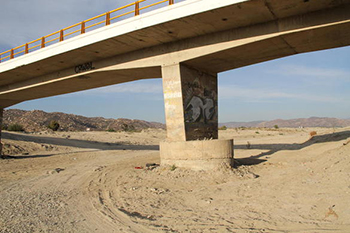online_contraction_scour_live_bed:  Contraction scour under live bed conditions
using modified Laursen 1960 equationModified Laursen formula

ys / y1 = ( Q2 / Q1 ) 0.86 (W1 / [W2) k1  -  ( yo / y1 )

[ yo, y1, Q and W in any consistent set of units ]
[ S1 in m/m or ft/ft ]
[ T in °C or °F ]
[ D50 in mm or ft ]

Reference:

 SAMPLE INPUT:      [Main Page]     [Description]

• Mean flow depth below the bridge, before scour yo =  1.0 m

• Mean flow depth upstream of the bridge y1 =  2.0 m/s

• Discharge upstream of the bridge (only in the main channel) Q1 =  10.0 m3/s

• Discharge in the contracted section Q2 =  8.0 m3/s

• Bottom width in the section upstream of the bridge W1 =  2.0 m

• Bottom width in the contracted section, excluding piers W2 =  1.0 m

• Energy slope in the main channel upstream S1 =  0.002 m/m

• Median diameter of the bed material D50 =  2.0 mm

• Water Temperature T =  20oC

SAMPLE OUTPUT

Contraction scour depth under live bed conditions ys =   1.57 m